# Tau method

(diff) ← Older revision | Latest revision (diff) | Newer revision → (diff)method

A method initially formulated as a tool for the approximation of special functions of mathematical physics (cf. also Special functions), which could be expressed in terms of simple differential equations. It developed into a powerful and accurate tool for the numerical solution of complex differential and functional equations. A main idea in it is to approximate the solution of a given problem by solving exactly an approximate problem.

## Lanczos' formulation of the tau method.

In [a17], C. Lanczos remarked that truncation of the series solution of a differential equation is, in some way, equivalent to introducing a perturbation term in the right-hand side of the equation. Conversely, a polynomial perturbation term can be used to produce a truncated series, that is, a polynomial solution.

Assume one wishes to solve by means of a power series expansion the simple linear differential equation (cf. also Linear differential operator)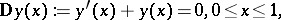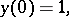which defines. To find the coefficients of a formal series expansion of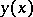, one substitutes the series in the equation and generates a system of algebraic equations for the coefficients: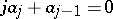for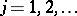, solving it in terms of. The value of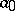is fixed using the initial condition. To find a finite expansion, say of order, one needs to make all coefficients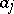with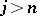equal to zero. This is achieved by adding a term of the form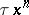to the right-hand side of the differential equation. One has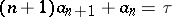, so that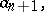and all the coefficients following it, will be equal to zero if one chooses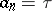. The same condition follows by substituting a segment of degreeof the series expansion of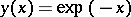into the equation. If the solution of the perturbed differential equation is regarded as an approximation to that of the original equation with, say, a right-hand side equal to zero, it seems natural to replace it by the best uniform approximation of zero over the same interval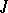, which is a Chebyshev polynomial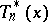of degree, defined over(cf. also Chebyshev polynomials).

Therefore, to find an accurate polynomial approximation of, Lanczos proposed solving exactly the more complex perturbed problem (the tau problem):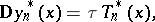with the same initial conditions as before. The polynomial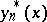is called the tau method approximation ofover the given interval.

This tau problem can be solved for the unknown coefficients ofusing several alternative procedures. One of them is described above, that is, to set up and solve a system of linear algebraic equations linking the unknown coefficients ofwith those of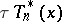. In this process one can assume thatitself can be expressed in either powers of, or in Chebyshev, Legendre or other polynomials. The first choice was Lanczos' original choice, and he explicitly indicated the possibility of choosing the others.

The second choice is a tau method, often [a8] called the Chebyshev method (or Legendre method) and, also, the spectral method. This last formulation of the tau method has been extensively used and applied, since 1971, to complex problems in fluid dynamics by S.A. Orsag [a11].

There are at least three other approaches to the tau method. One of them is to find the coefficients of the approximant through a process of interpolation at the zeros of the perturbation term. This early form of collocation was termed the "method of selected points" by Lanczos [a17]. When the perturbation term is an orthogonal polynomial (such as a Chebyshev, Legendre, or other polynomial), this process is called "orthogonal collocation" . This is the name by which Lanczos' method of selected points is usually designated today (as of 2000); the name "pseudo-spectral method" is also often applied to it. Algorithms for these methods have been well developed.

## Recursive formulation of the tau method based on canonical polynomials.

In his classic [a18], Lanczos noted that if a sequence of polynomials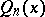,, such thatfor all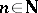can be found for any linear differential operator with polynomial coefficients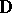, then, since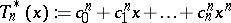(the coefficients of which are tabulated), the solution of the tau problem would be immediately given by: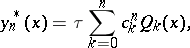where the parameteris fixed using the initial condition.

An extension of this approach to a wider range of differential operators than the trivial one, given in the example, has several advantages: canonical polynomials are independent of the interval in which the solution is sought, allowing for easy segmentation of the domain; they are permanent, in the sense that if an approximation of a higher degree is required, the computation does not need to be repeated from scratch; they are also independent of the supplementary conditions of the problem, which can now equally be initial, boundary or multi-point conditions. Furthermore, the tau method does not require a stage of discretization of the given differential operator, as discrete-variable methods do.

A sequence of canonical polynomials defined as simply asfor all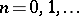, need not always exist or need not be unique. An algebraic and algorithmic theory of the tau method, initially constructed for elementsof the class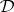of linear differential operators of arbitrary integer order, with polynomial or rational coefficients (essentially the tools a computer handles) was discussed by E.L. Ortiz in [a24]. In this work, canonical polynomials are defined as realizations of classes of equivalence of polynomials, for which the algebraic kernel of the differential operator is the modulus. These classes have gaps in their index sequence. Elements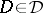are then uniquely associated with representatives of such classes of canonical sequences. The codimension of the image of the space of polynomials under operatorsis usually small, and bounded by the order ofplus the height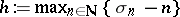(where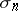is the degree of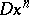) of the differential operator.

For more general operators than the one used as an example, more than a singleterm is usually required to satisfy the more elaborate supplementary conditions and, also, internal conditions of the method. In the case of a problem defined by a differential operatorin, of orderand with non-constant coefficients, the question of the number ofterms required for a tau method approximation has been shown to be related to the size of the gap in the canonical sequence, and to the existence of a non-empty algebraic kernel in. The number ofterms can be easily determined in this approach using information on the degree of polynomial (or rational) coefficients and the order of differentiation of the function to which they apply. It was also shown in [a24] that canonical sequences can be generated recursively. This approach was used to formulate the first recursive algorithms for the automatic solution of differential equations using the tau method. The theory of canonical polynomials has been discussed and extended by several authors; see [a10] and the references given therein.

Theoretical error analysis for the tau method [a18], [a30], [a9], [a22], [a26] have shown that tau method approximations are of the order of best uniform approximations by polynomials defined over the same interval. This connection with best approximation is preserved when a tau method based on rational approximation [a18], [a21] is used [a6].

## Operational formulation of the tau method.

There is yet another way in which tau method approximations can be constructed. An operational formulation of the tau method was introduced by Ortiz and H. Samara in [a27]. In this formulation, derivatives and polynomial coefficients of operators inare represented in terms of multiplicative diagonal matrices. Furthermore, the differential operator and the supplementary conditions are decoupled. Through a simple and systematic algorithm, which treats the differential operator and supplementary conditions with similar machinery, this technique transforms a given differential tau method problem into one in linear algebra. The approximate solution can be generated, indistinctively, in terms of powers of the variables or in terms of elements of a more stable polynomial basis, such as Chebyshev, Legendre or other polynomials. The operational formulation further simplified the development of software for the tau method.

## Numerical applications of the tau method.

The recursive and operational approaches to the tau method have been extended in several directions. To systems of linear differential equations [a9], [a4]; to non-linear problems [a25], [a23], [a26]; to partial differential equations [a28], [a29]; and, in particular, to the numerical solution of non-linear systems of partial differential equations the solution of which has sharp spikes, with high gradients, as in the case of soliton interactions [a13], [a14]; to the approximate solution of ordinary and partial functional-differential equations [a25], [a20], [a16]; and to singular problems for partial differential equations related to crack propagation [a7]. The tau method is well adapted to produce accurate approximations in the numerical treatment of differential eigenvalue problems with one or multiple spectral parameters, entering either linear or non-linearly into the equation [a2], [a19]. The tau method has been extensively used for the high-precision approximation of real- [a15] and complex-valued functions. A weak formulation of the tau method has been proposed and applied to inverse problems for partial differential equations [a1].

## Analytical applications of the tau method.

The tau method has also been used in a totally different direction, as a tool in the discussion of problems in mathematical analysis, for example, in complex function theory [a12].

Possible connections between the tau method, collocation, Galerkin's method, algebraic kernel methods, and other polynomial or discrete-variable techniques have also been explored [a31], [a14], [a5].

The tau method has also received some attention as an analytic tool in the discussion of equivalence results across numerical methods [a5]. It has been found that, with it, it is possible to construct special "tau methods" , which recursively generate solutions numerically identical to those of collocation, Galerkin's and other weighted residual methods, and to those of discrete-variable methods, such as sophisticated forms of Runge–Kutta methods. This work suggests a way of unifying a large group of continuous- and discrete-variable approximation techniques.

How to Cite This Entry:
Tau method. Encyclopedia of Mathematics. URL: http://encyclopediaofmath.org/index.php?title=Tau_method&oldid=18668
This article was adapted from an original article by Eduardo L. Ortiz (originator), which appeared in Encyclopedia of Mathematics - ISBN 1402006098. See original article